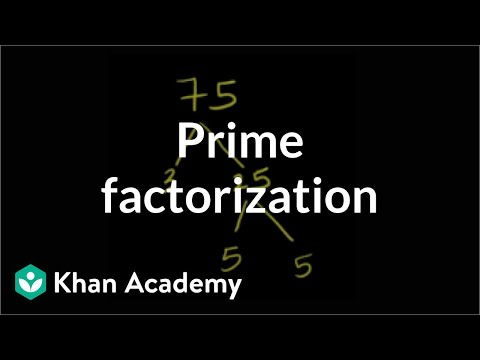## Algebraic Expresssion

Subject: Compulsory Maths

Find Your Query

#### Overview

Factorization is the process of expressing the given algebraic expression as the product of two or more algebraic expressions or terms.

##### Algebraic Expresssion### Factorization

Factorization is the process of expressing the given algebraic expression as the product of two or more algebraic expression or terms. For example, 2x+5x2  = x(2 + 5x) can be expressed as the product of x and 2 +5x.i.e 2x+52 = x(2 + 5x).When an algebraic expression is the product of two or more expressions, each of these expressions is called a factor of a given expression.In the above examples, x and 2x+5x are the factors of 2x+5x2.

Factorization of the expression of the form a2-b2

(i) Take a square piece of paper with one side 'a' unit whose area becomes as sq.units.

(ii) In one of the corners of the square, cut off a small square of side 'b' units. The area of this small squares is so units.

(iii) Let's cut a2-b2 diagonally as shown in the figure.(iv) Arrange two parts to make a rectangle.

Length of the rectangle = (a+b) units

Breagth of the rectangle = (a-b)

Now, area of the rectangle = length × breadth

= (a+b)(a-b)

= a2-b2

∴ Area of rectangle = (a2-b2) sq.unit

∴ a2-b2 = (a+b)(a-b)

##### Things to remember

Some useful formula

• (a+b)= a2+2ab+b2 or (a+b)2+4ab
• (a-b)2 = a2-2ab+b2 or, (a+b)2-4ab
• a2-b= (a+b)(a-b)
• a2+b2 = (a+b)2-2ab or, (a-b)2+2ab
• It includes every relationship which established among the people.
• There can be more than one community in a society. Community smaller than society.
• It is a network of social relationships which cannot see or touched.
• common interests and common objectives are not necessary for society.
##### Videos for Algebraic Expresssion##### Math Antics - Prime Factorization##### Math- How to factor- Math Help How to- Factoring##### Questions and Answers

Soln:16x4-4x2

= 4x2(4x2-1)

= 4x2{(2x2)(1)2}

=4x2(2x+1)(2x-1) Ans.

Soln:3x3-48x

= 3x(x2-16)

= 3x(x2-42)

=3x(x+4)(x-4) Ans.

Soln: a4-b4

=(a2)2-(a2)2-(b2)2

=(a2+b2)(a2-b2)

=(a2+b2)(a+b)(a-b) Ans.

Soln:(y+1)2-4

=(y+1)2-(2)2

=(y+1+2)(y+1-2)

= (y+3)(y-1)Ans.

Soln:36-(x+5)2

= 62-(x+5)2

=(6+x+5)(6-x-5)

=(11+x)(1-x) Ans.

Soln:a2+2ab+b2-c2

= (a+b)2-c2

=(a+b+c)(a+b-c) Ans.

Soln: m2+n2-2mn-p2

=m2-2mn+n2-p2

=(m -n)2-p2

= (m-n+p)(m-n-p) Ans.

Soln:ab2-b(a-c)-c

= ab2-ab+bc-c

= ab(b-1) +c (b-1)

= (b-1)(ab+c) Ans.

Soln:x2-2(x+y)-y2

= x2-y2-2(x+y)

= (x+y)(x-y)-2(x+y)

=(x+y)(x-y-2) Ans.

Soln:(32)2-(28)2

= (32+28)(32-28)

= 60 ×4

= 240 Ans.

Soln:(51)2 -(49)2

= (51+49)(51-49)

= 100× 2

= 200 Ans.

Soln: (101)2-(99)2

= (101+99)(101-99)

= 200 × 2

=400 Ans.

Soln:(7.9)2-(2.1)2

=(7.9)2-(2.1)2

=(7.9 +2.1)(7.9-2.1)

= 10.0 × 5.8

= 58 Ans

Soln: 4x2+9x+5

= 4x2+5x +4x+5

= 4x2+5x+4x+5

= x(4x+5)+1(4x+5)

=(x+1)(4x+5) Ans.

Soln:4x2-12x+5

= 4x2-10x -2x+5

= 2x(2x-5)-1(2x-5)

=(2x-1)(2x-5) Ans.

Soln:p4+4

=(p2)2+22

=(p2)2+2.p2.2+22-4p2

=(p2+2)2-4p2=(p2+2)2-(2p)2

=(p2+2+2p)(p2+2-2p)

=(p2+2p+2)(p2-2p+2) Ans.

Soln:a4+4

=(a2)2+22

=(a2)2+2.a2.2+22-4a52

=(a2+2)2-4a2=(a2+2)2-(2a)2

=(a2+2+2a)(a2+2-2a)

=(a2+2a+2)(a2-2a+2) Ans.

##### Quiz

© 2019-20 Kullabs. All Rights Reserved.